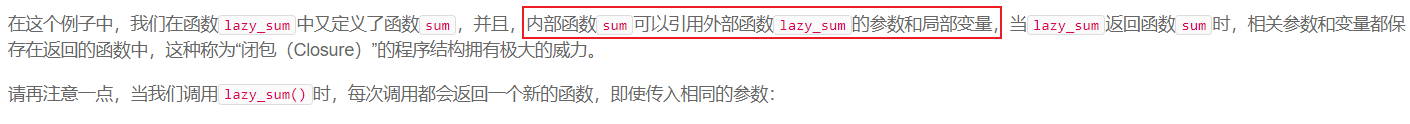# 闭包变量引用def aa():
x = 0

def bb():
x = 1
return x
return counter

aa函数里的x变量，是属于aa()定义域里的变量，这里的x指向了0这个int对象。而在bb()函数里的x变量，是bb()定义域里的变量，这里的x则指向了1这个int对象。

• 使用一个可变参数，如字典，列表，使用append方法和pop方法增删值
• 在内部方法使用nonlocal关键字声明这个变量指向非本地对象，然后变量就会向上查询对象。

def cc():
x = 0
y = [1, 2]

def dd():
nonlocal x
x += 1
y.append(3)
return x, y
return dd

cc()
ee = cc()
print(ee())

# 闭包到底是啥

def counter():
x = 0
# 这里检测counter()作用域中x的值
print(x, end=',')
# 改变一下x的值
x += 1
print(x, end=',')

def count_counter():
nonlocal x
x += 1
# 这里检测count_counter()作用域中x的值
print(x, end=',')
return x

return count_counter

# 连续三遍执行程序，查看输出的值
a = counter()
# 先执行三遍counter()
counter(), counter(), counter()
# 再执行三遍count_counter()
a(), a(), a()

0,1,0,1,0,1,0,1,2,3,4,

• 分类：
• 标签：

### 2 条评论

1.##### 王木木 · 2020-08-03 22:59

放心吧,闭包这个东西以后99.999的概率用不上,java 还支持闭包写法但是有几个人听说过

1.##### avimitin · 2020-08-10 21:18 作者

当时看着黑马学的，所以就（（